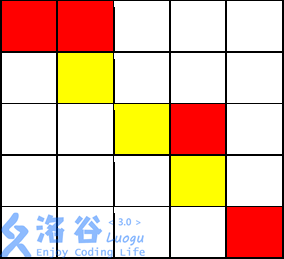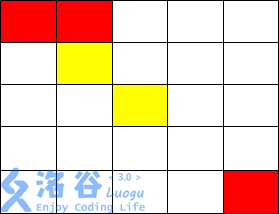# 题面

NOIP2017 普及组 T3

## 提示

### 输入输出样例 1 说明$(1,1)$开始，走到$(1,2)$不花费金币

$(1,2)$向下走到$(2,2)$花费 $1$ 枚金币

$(2,2)$施展魔法，将$(2,3)$变为黄色，花费 $2$ 枚金币

$(2,2)$走到$(2,3)$不花费金币

$(2,3)$走到$(3,3)$不花费金币

$(3,3)$走到$(3,4)$花费 $1$ 枚金币

$(3,4)$走到$(4,4)$花费 $1$ 枚金币

$(4,4)$施展魔法，将$(4,5)$变为黄色，花费$2$ 枚金币，

$(4,4)$走到$(4,5)$不花费金币

$(4,5)$走到$(5,5)$花费 $1$ 枚金币

### 输入输出样例 2 说明$( 1, 1)$走到$( 1, 2)$,不花费金币

$( 1, 2)$走到$( 2, 2)$,花费$1$金币

$( 2, 3)$走到$( 3, 3)$不花费金币

$( 3, 3)$只能施展魔法到达$( 3, 2),( 2, 3),( 3, 4),( 4, 3)$

# 思路

## 怎么走

x,y表示坐标，coin表示当前的金币数量，color表示当前坐标的颜色，can表示当前是否能施展魔法。

dfs(nx,ny,coin,1,color);

dfs(nx,ny,coin+1,1,mp[nx][ny]);

dfs(nx,ny,coin+2,0,color);## 剪枝1. 发现当前金币已经比最小的多了，就不用搜下去了
2. 在循环中就可以判断搜索合法性了，减小函数调用开销（PS：我习惯把这玩意写在边界条件上面，看起来差不多，实则增加了函数调用开销，调用后才返回，花费显然很大
3. 记忆化，记录走到当前坐标合法路径的最小金币，如果超过就可以不搜了，比第一个更高级